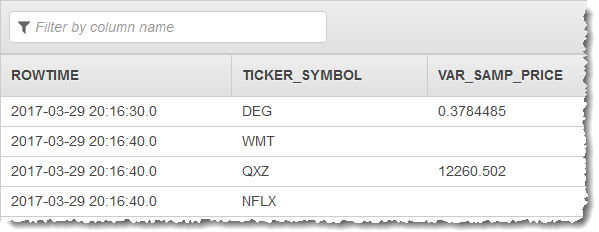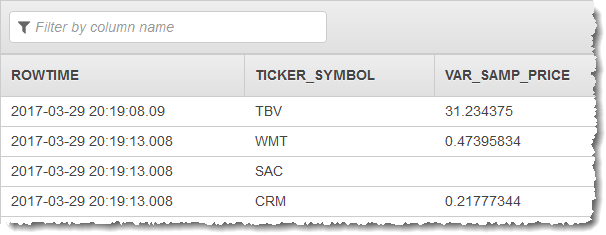VAR_SAMP - Amazon Kinesis Data Analytics
Services or capabilities described in Amazon Web Services documentation might vary by Region. To see the differences applicable to the China Regions, see Getting Started with Amazon Web Services in China.

# VAR_SAMP

Returns the sample variance of a non-null set of numbers (nulls being ignored).

VAR_SAMP uses the following calculation:

• (SUM(expr*expr) - SUM(expr)*SUM(expr) / COUNT(expr)) / (COUNT(expr)-1)

In other words, for a given set of non-null values, using S1 as the sum of the values and S2 as the sum of the squares of the values, `VAR_SAMP` returns the result (S2-S1*S1/N)/(N-1).

When you use `VAR_SAMP`, be aware of the following:

• When the input set has no non-null data, `VAR_SAMP` returns `NULL`. Given an input set of null or one element, `VAR_SAMP` returns `null`.

• If you don't use the `OVER` clause, `VAR_SAMP` is calculated as an aggregate function. In this case, the aggregate query must contain a GROUP BY clause on a monotonic expression based on `ROWTIME` that groups the stream into finite rows. Otherwise, the group is the infinite stream, and the query will never complete and no rows will be emitted. For more information, see Aggregate Functions.

• A windowed query that uses a `GROUP BY` clause processes rows in a tumbling window. For more information, see Tumbling Windows (Aggregations Using GROUP BY).

• If you use the `OVER` clause, `VAR_SAMP` is calculated as an analytic function. For more information, see Analytic Functions.

• A windowed query that uses an `OVER` clause processes rows in a sliding window. For more information, see Sliding Windows

## Syntax

`````` VAR_SAMP ( [DISTINCT | ALL] number-expression )
``````

## Parameters

### ALL

Includes duplicate values in the input set. `ALL` is the default.

### DISTINCT

Excludes duplicate values in the input set.

## Examples

### Example Dataset

The examples following are based on the sample stock dataset that is part of the Getting Started Exercise in the Amazon Kinesis Analytics Developer Guide. To run each example, you need an Amazon Kinesis Analytics application that has the sample stock ticker input stream. To learn how to create an Analytics application and configure the sample stock ticker input stream, see Getting Started in the Amazon Kinesis Analytics Developer Guide.

The sample stock dataset has the schema following.

``````
(ticker_symbol  VARCHAR(4),
sector          VARCHAR(16),
change          REAL,
price           REAL)
``````

### Example 1: Determine the sample variance in a column in a tumbling window query

The following example demonstrates how to use the `VAR_SAMP` function to determine the sample variance of the values in a tumbling window of the PRICE column of the example dataset. `DISTINCT` is not specified, so duplicate values are included in the calculation.

#### Using STEP (Recommended)

``````CREATE OR REPLACE STREAM "DESTINATION_SQL_STREAM" (ticker_symbol VARCHAR(4), var_samp_price REAL);

CREATE OR REPLACE  PUMP "STREAM_PUMP" AS INSERT INTO "DESTINATION_SQL_STREAM"

SELECT STREAM ticker_symbol, VAR_SAMP(price) AS var_samp_price
FROM "SOURCE_SQL_STREAM_001"
GROUP BY ticker_symbol, STEP(("SOURCE_SQL_STREAM_001".ROWTIME) BY INTERVAL '60' SECOND);
``````

#### Using FLOOR

``````CREATE OR REPLACE STREAM "DESTINATION_SQL_STREAM" (ticker_symbol VARCHAR(4), var_samp_price REAL);

CREATE OR REPLACE  PUMP "STREAM_PUMP" AS INSERT INTO "DESTINATION_SQL_STREAM"

SELECT STREAM ticker_symbol, VAR_SAMP(price) AS var_samp_price
FROM "SOURCE_SQL_STREAM_001"
GROUP BY ticker_symbol, FLOOR(("SOURCE_SQL_STREAM_001".ROWTIME - TIMESTAMP '1970-01-01 00:00:00') SECOND / 10 TO SECOND);
``````

#### Results

The preceding examples output a stream similar to the following:### Example 2: Determine the sample variance of the values in a column in a sliding window query

The following example demonstrates how to use the `VAR_SAMP` function to determine the sample variance of the values in a sliding window of the PRICE column of the example dataset. `DISTINCT` is not specified, so duplicate values are included in the calculation.

``````CREATE OR REPLACE STREAM "DESTINATION_SQL_STREAM" (ticker_symbol VARCHAR(4), var_samp_price REAL);

CREATE OR REPLACE PUMP "STREAM_PUMP" AS INSERT INTO "DESTINATION_SQL_STREAM"

SELECT STREAM ticker_symbol, VAR_SAMP(price) OVER TEN_SECOND_SLIDING_WINDOW AS var_samp_price
FROM "SOURCE_SQL_STREAM_001"

WINDOW TEN_SECOND_SLIDING_WINDOW AS (
PARTITION BY ticker_symbol
RANGE INTERVAL '10' SECOND PRECEDING);
``````

The preceding example outputs a stream similar to the following: